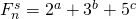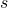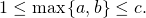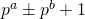# s-th power of Fibonacci number of the form 2a + 3b + 5c

Nurettin Irmak and Bo He
Notes on Number Theory and Discrete Mathematics
Print ISSN 1310–5132, Online ISSN 2367–8275
Volume 25, 2019, Number 4, Pages 102–109
DOI: 10.7546/nntdm.2019.25.4.102-109

## Details

### Authors and affiliations

Nurettin IrmakDepartment of Mathematics, Art and Science Faculty,
Niğde Ömer Halisdemir University, Turkey

Bo HeInstitute of Mathematics, Aba Teachers University
Wenchuan, Sichuan, 623000 P. R. China

### Abstract

In this paper, we solve the Diophantine equation, whereandare positive integers with### Keywords

• Fibonacci numbers
• Linear forms in logarithms
• Reduction method
• s-th power

• 11B39
• 11J86

### References

1. Bertók, C., Hajdu, L., Pink, I. & Rábai, Z. (2017). Linear combinations of prime powers in binary recurrence sequences. Int. J. Number Theory, 13 (2), 261–271.
2. Bugeaud, Y., Mignotte, M., & Siksek, S. (2006). Classical and modular approaches to exponential Diophantine equation. I. Fibonacci and Lucas perfect powers. Ann of Math, 163, 969–1018.
3. Bravo, J. J., & Luca, F. (2012). Powers of two in generalized Fibonacci sequence.Rev Colombiana Math, 46, 67–79.
4. Luca, F. & Szalay, L. (2007). Fibonacci numbers of the form. The Fibonacci Quarterly, 45, 98–103.
5. Marques, D. & Togbé, A. (2013). Fibonacci and Lucas numbers of the formProc Japan Acad, 89, 47–50.
6. Matveev, E. M. (2000). An explicit lower bound for a homogeneous linear form in logarithms of algebraic numbers. II, Izv Ross Akad Nauk Ser Mat, 64 (6), 125–180.
7. Koshy, T. (2001). Fibonacci and Lucas Numbers with Applications, USA: Wiley.
8. Luca, F. & Oyono, R. (2011). An exponential Diophantine equation related to powers of two consecutive Fibonacci numbers. Proc Japan Acad., 87 (A), 45–50.
9. Shorey, T. N. & Stewart, C. L. (1987). Pure powers in recurrence sequences and some related Diophantine equations. J. Number Theory, 27 (3), 324–352.

## Cite this paper

Irmak, N., & He, B. (2019). s-th power of Fibonacci number of the form 2a + 3b + 5c. Notes on Number Theory and Discrete Mathematics, 25(4), 102-109, doi: 10.7546/nntdm.2019.25.4.102-109.